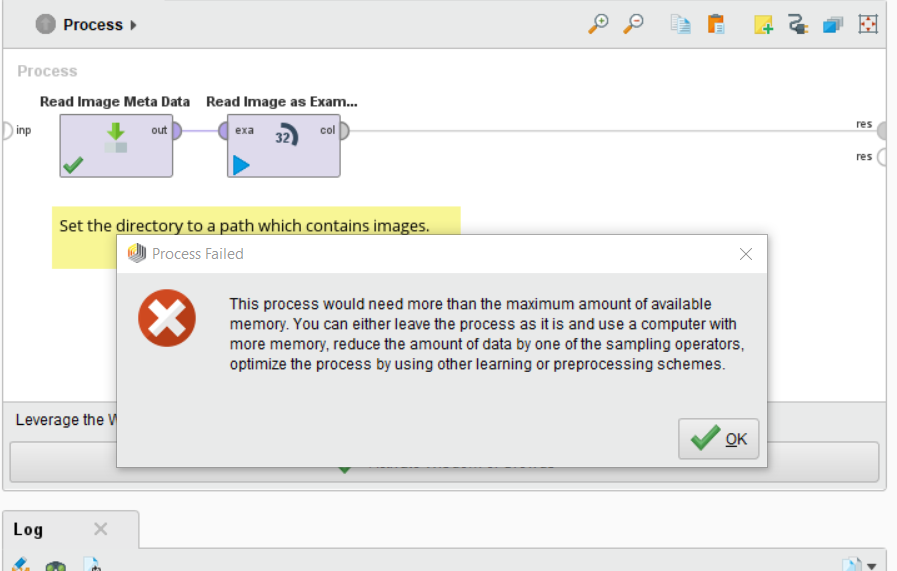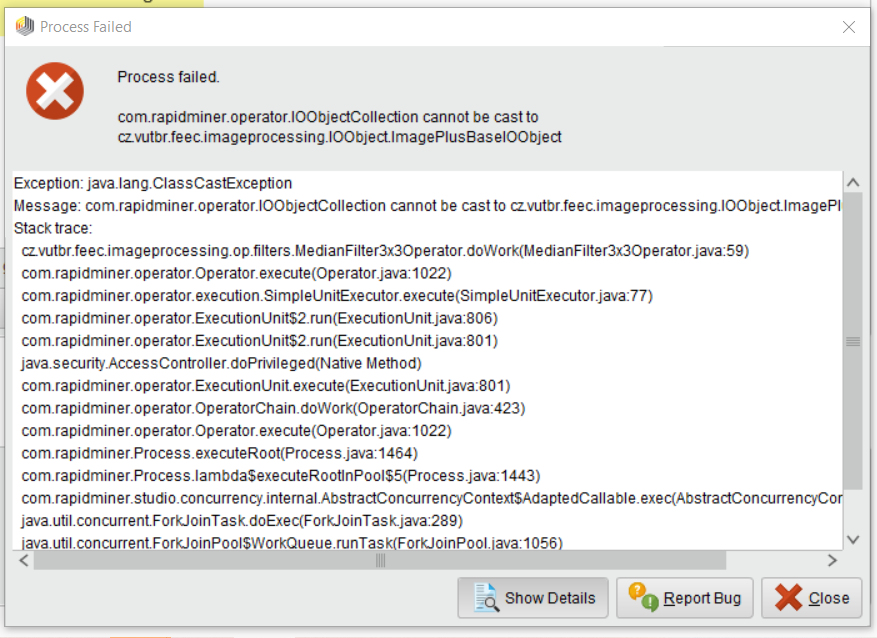# How to extract the RGB color composition of an image in value?

Member Posts: 10Newbie
I would like to extract the value percentage of each red, blue and green percentage of the image and put it as an attribute in database.

• Member, University Professor Posts: 391Unicorn
Solution Accepted
Install "Image Handling" extension, and there "Read Image as ExampleSet" will read the directory of images, and represent each image as an RGB tripple of values in the range 0..255.
• Member, University Professor Posts: 391Unicorn
Solution Accepted
Try with one low resolution image, eg PNG, and see what happens.

• Member Posts: 10Newbie
edited December 2020
Do it has limit to process the image? my file only contained 46 images. What should I do to overcome this problem?• Member Posts: 10Newbie
Thank you for your help, but the result is still not transfer into attribute database. What operator should I used so that these value can be nicely stored in attribute dataset?
• Member, University Professor Posts: 391Unicorn
Each picture is represented as three sets of numbers c columns time r rows of Red, Green and Blue pixel values. The question is now how would you like to store these away? You may need to convert them into into some flat structure perhaps, which may be challenging if your pictures are of different size.
• Member Posts: 10Newbie
edited January 2021
I would like to use Median Filter but I am having problem  the example set is a huge data. How can I fit this data into Median Filter?• Member, University Professor Posts: 391Unicorn
I am not sure what you wish to do with these captured images, if you want to keep color or not, or if the images have or have not been previously scaled. Here is an quick example of how you can capture a directory full of images. I read all image meta information first. Then I read these images in grayscale (one channel only), which returns a collection of channel collections (in our case one only per image). The collection of images can now be flattened but not yet merged as each image is of a different size. So I process each image in a loop over the collection and for each select only 100 rows and 100 columns (essentially it is a crop), I also add an attribute to identify each image by its number, which later I'll define as a batch type. Now all image information can be appended and stored if wished to.

```<?xml version="1.0" encoding="UTF-8"?><process version="9.8.001">
<context>
<input/>
<output/>
<macros/>
</context>
<operator activated="true" class="process" compatibility="9.8.001" expanded="true" name="Process" origin="GENERATED_TUTORIAL">
<parameter key="logverbosity" value="init"/>
<parameter key="random_seed" value="2001"/>
<parameter key="send_mail" value="never"/>
<parameter key="process_duration_for_mail" value="30"/>
<parameter key="encoding" value="SYSTEM"/>
<process expanded="true">
<operator activated="true" class="image_handling:read_image_meta_data" compatibility="0.2.001" expanded="true" height="68" name="Read Image Meta Data" origin="GENERATED_TUTORIAL" width="90" x="45" y="34">
<parameter key="directory" value="/home/jacob/Pictures/Wallpapers"/>
<parameter key="use_label" value="false"/>
</operator>
<operator activated="true" class="image_handling:read_image_as_exampleset" compatibility="0.2.001" expanded="true" height="68" name="Read Image as ExampleSet" origin="GENERATED_TUTORIAL" width="90" x="179" y="34">
<parameter key="path" value="Path"/>
<parameter key="height" value="Height"/>
<parameter key="width" value="Width"/>
<parameter key="number_of_color_channels" value="1"/>
</operator>
<operator activated="true" class="flatten_collection" compatibility="9.8.001" expanded="true" height="68" name="Flatten Collection" width="90" x="313" y="34"/>
<operator activated="true" class="loop_collection" compatibility="9.8.001" expanded="true" height="82" name="Loop Collection" width="90" x="447" y="34">
<parameter key="set_iteration_macro" value="true"/>
<parameter key="macro_name" value="iteration"/>
<parameter key="macro_start_value" value="1"/>
<parameter key="unfold" value="false"/>
<process expanded="true">
<operator activated="true" class="extract_macro" compatibility="9.8.001" expanded="true" height="68" name="Extract Macro" width="90" x="179" y="34">
<parameter key="macro" value="last_attr"/>
<parameter key="macro_type" value="number_of_attributes"/>
<parameter key="statistics" value="average"/>
<parameter key="attribute_name" value=""/>
</operator>
<operator activated="true" class="filter_example_range" compatibility="9.8.001" expanded="true" height="82" name="Filter Example Range" width="90" x="313" y="34">
<parameter key="first_example" value="1"/>
<parameter key="last_example" value="100"/>
<parameter key="invert_filter" value="false"/>
</operator>
<operator activated="true" class="remove_attribute_range" compatibility="9.8.001" expanded="true" height="82" name="Remove Attribute Range" width="90" x="447" y="34">
<parameter key="first_attribute" value="101"/>
<parameter key="last_attribute" value="%{last_attr}"/>
</operator>
<operator activated="true" class="generate_attributes" compatibility="9.8.001" expanded="true" height="82" name="Generate Attributes" width="90" x="581" y="34">
<list key="function_descriptions">
<parameter key="imageno" value="%{iteration}"/>
</list>
<parameter key="keep_all" value="true"/>
</operator>
<connect from_port="single" to_op="Extract Macro" to_port="example set"/>
<connect from_op="Extract Macro" from_port="example set" to_op="Filter Example Range" to_port="example set input"/>
<connect from_op="Filter Example Range" from_port="example set output" to_op="Remove Attribute Range" to_port="example set input"/>
<connect from_op="Remove Attribute Range" from_port="example set output" to_op="Generate Attributes" to_port="example set input"/>
<connect from_op="Generate Attributes" from_port="example set output" to_port="output 1"/>
<portSpacing port="source_single" spacing="0"/>
<portSpacing port="sink_output 1" spacing="0"/>
<portSpacing port="sink_output 2" spacing="0"/>
</process>
</operator>
<operator activated="true" class="append" compatibility="9.8.001" expanded="true" height="82" name="Append" width="90" x="581" y="34">
<parameter key="datamanagement" value="double_array"/>
<parameter key="data_management" value="auto"/>
<parameter key="merge_type" value="all"/>
</operator>
<operator activated="true" class="set_role" compatibility="9.8.001" expanded="true" height="82" name="Set Role" width="90" x="715" y="34">
<parameter key="attribute_name" value="imageno"/>
<parameter key="target_role" value="batch"/>
</operator>
<connect from_op="Read Image Meta Data" from_port="output" to_op="Read Image as ExampleSet" to_port="example set"/>
<connect from_op="Read Image as ExampleSet" from_port="collection" to_op="Flatten Collection" to_port="collection"/>
<connect from_op="Flatten Collection" from_port="flat" to_op="Loop Collection" to_port="collection"/>
<connect from_op="Loop Collection" from_port="output 1" to_op="Append" to_port="example set 1"/>
<connect from_op="Append" from_port="merged set" to_op="Set Role" to_port="example set input"/>
<connect from_op="Set Role" from_port="example set output" to_port="result 1"/>
<portSpacing port="source_input 1" spacing="0"/>
<portSpacing port="sink_result 1" spacing="0"/>
<portSpacing port="sink_result 2" spacing="0"/>
<description align="left" color="yellow" colored="false" height="50" resized="true" width="327" x="39" y="126">Set the directory to a path which contains images.</description>
</process>
</operator>
</process>
```

• Member, University Professor Posts: 391Unicorn
If you wanted to work with very large number of high resolution images, It is probably best to pre-process then first outside RapidMiner. Python has a great range image processing libraries, e.g. see: https://towardsdatascience.com/image-manipulation-tools-for-python-6eb0908ed61f